New update is available. Click here to update.
Topics

# Shortest path in an unweighted graph

Moderate0/80
Average time to solve is 25m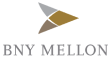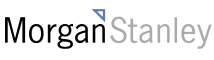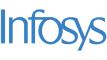+15 more companies

## Problem statement

The city of Ninjaland is analogous to the unweighted graph. The city has ‘N’ houses numbered from 1 to ‘N’ respectively and are connected by M bidirectional roads. If a road is connecting two houses ‘X’ and ‘Y’ which means you can go from ‘X’ to ‘Y’ or ‘Y’ to ‘X’. It is guaranteed that you can reach any house from any other house via some combination of roads. Two houses are directly connected by at max one road.

A path between house ‘S’ to house ‘T’ is defined as a sequence of vertices from ‘S’ to ‘T’. Where starting house is ‘S’ and the ending house is ‘T’ and there is a road connecting two consecutive houses. Basically, the path looks like this: (S , h1 , h2 , h3 , ... T). you have to find the shortest path from ‘S’ to ‘T’.

For example
``````In the below map of Ninjaland let say you want to go from S=1 to T=8, the shortest path is (1, 3, 8). You can also go from S=1 to T=8  via (1, 2, 5, 8)  or (1, 4, 6, 7, 8) but these paths are not shortest.
``````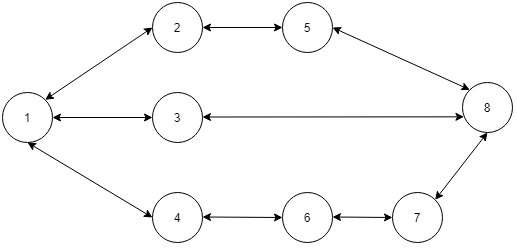Detailed explanation ( Input/output format, Notes, Images )
Constraints :
``````1 <= T <= 100
2 <= N <= 10 ^ 3
1 <= M <= min( N *(N - 1) / 2 , 1000 )
1 <= S, T <= N

Time Limit: 1 sec
``````
Sample input 1 :
``````1
4 4
1 4
1 2
2 3
3 4
1 3
``````
Sample Output 1 :
``````( 1 , 3 , 4 )
``````
Explanation of Sample input 1 :
``````In the above graph there are two ways to go from 1 to 4 ,
( 1 , 2 , 3 , 4 ) and ( 1 , 3 , 4 ) but the second path is the shortest path.
``````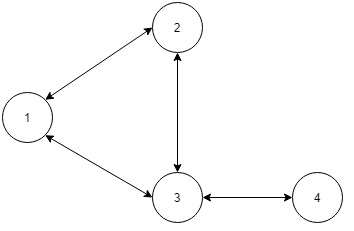Sample input 2 :
``````1
8 9
1 8
1 2
1 3
1 4
2 5
5 8
3 8
4 6
6 7
7 8
``````
Sample output 2 :
``````( 1 , 3 , 8 )
``````Console